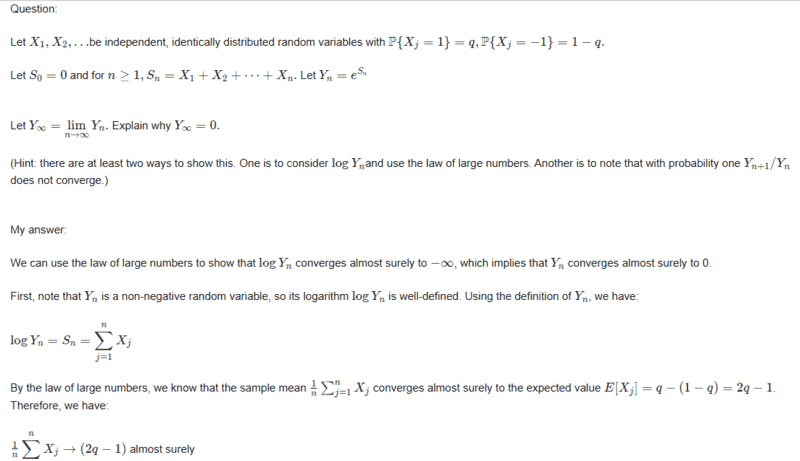# Show that ##Y_{\infty}=0 ##

• I
• WMDhamnekar

#### WMDhamnekar

MHB
Let ##X_1, X_2, \dots ##be independent, identically distributed random variables with

##P{X_j = 1} = q, P{X_j = −1} = 1 − q.##

Let ##S_0 = 0 ##and for n ≥ 1, ##S_n = X_1 + X_2 + \dots + X_n.## Let ##Y_n = e^{S_n}##
Let ##Y_{\infty} = \lim\limits_{n\to\infty} Y_n.## Explain why ##Y_{\infty} = 0.## (Hint: there are at least two ways to show this. One is to consider ##\log{Y_n}## and use the law of large numbers. Another is to note that with probability one ##Y_{n+1}/Y_n## does not converge.)

We can use the law of large numbers to show that ##\log Y_n## converges almost surely to ##-\infty##, which implies that ##Y_n## converges almost surely to 0.

First, note that ##Y_n## is a non-negative random variable, so its logarithm ##\log Y_n## is well-defined. Using the definition of ##Y_n##, we have:

##\log{Y_n}= S_n =\displaystyle\sum_{j=1}^n X_j##

By the law of large numbers, we know that the sample mean $\frac{1}{n}\sum_{j=1}^{n}X_j$ converges almost surely to the expected value ##E[X_j] = q - (1-q) = 2q-1.## Therefore, we have:

##\frac{1}{n}\displaystyle\sum_{j=1}^n X_j \to (2q-1)## almost surely

Using this result, we can show that ##\log{Y_n}## converges almost surely to ##-\infty## as follows:

####\begin{align*}
\log Y_n &= \sum_{j=1}^{n} X_j \ \
&= n\cdot\frac{1}{n}\sum_{j=1}^{n} X_j \ \
&\to n(2q-1) \quad \text{almost surely} \ \
&= \infty \quad \text{if } q > \frac{1}{2} \ \
&= -\infty \quad \text{if } q < \frac{1}{2}
\end{align*}####

When ##q < \frac{1}{2}##, we have ##\log{Y_n} \to -\infty## almost surely, which implies that ##Y_{\infty} = 0## almost surely.

Alternatively, we can use the fact that ##Y_n## is a martingale to show that ##Y_n## does not converge almost surely. By definition, ##Y_n## is a martingale because ##E[Y_{n+1} \mid Y_1,\ldots,Y_n] = E[e^{S_{n+1}} \mid Y_1,\ldots,Y_n] = e^{S_n} = Y_n##. Using the martingale convergence theorem, we know that if ##Y_n## converges almost surely to a limit ##Y_{\infty}##, then ##Y_{\infty}## must be a constant because ##Y_n## is a martingale. However, we can show that ##Y_{n+1}/Y_n## does not converge almost surely, which implies that ##Y_n## does not converge almost surely.

To see why ##Y_{n+1}/Y_n## does not converge almost surely, note that:

####\begin{align*}\displaystyle\frac{Y_{n+1}}{Y_n} &= \displaystyle\frac{e^{S_{n+1}}}{e^{S_n}} \ \
&= e^{S_{n+1}-S_n} \ \
&= e^{X_{n+1}}

\end{align*}####

Since ##X_{n+1}## takes on the values 1 and -1 with positive probability, we have ##e^{X_{n+1}} = e## or ##1/e## with positive probability. Therefore, ##Y_{n+1}/Y_n## does not converge almost surely, which implies that ##Y_n ##does not converge almost surely.

If ##q = 1/2##, then ##X_j## is a symmetric random variable with ##E[X_j] = 0##. In this case, ##S_n## is a random walk with mean zero, which means that ##\log{Y_n} = S_n## also has mean zero. By the law of large numbers, we know that ##\frac{1}{n}\cdot S_n## converges almost surely to zero, which implies that ##S_n## converges with probability one to zero. Using the continuous mapping theorem, we have ##\log{Y_n} \to 0## with probability one, which implies that ## Y_n \to 1##with probability one. Therefore, ##Y_n## converges to 1 with probability one when ##q = 1/2##.

Last edited:

Any reason it shouldn't be ?

Where's my magnifier ?

##\ ##

Any reason it shouldn't be ?

Where's my magnifier ?

##\ ##
Please open images in new tabs and zoom them in 125% or larger than 125%. No need for magnifier.Please open images in new tabs and zoom them in 125% or larger than 125%. No need for magnifier.DId just that and full is my screen with just the first few lines. But already doubt creeps in:
Any limitations on ##q## ? If not, for ##q=1## disaster looms ...

Or did my magnifier disrupt something ?

##\ ##

••WMDhamnekar and FactChecker
DId just that and full is my screen with just the first few lines. But already doubt creeps in:
Any limitations on ##q## ? If not, for ##q=1## disaster looms ...

Or did my magnifier disrupt something ?

##\ ##
I have written the answer in LaTeX form now.I have written the answer in LaTeX form now.But the question is lost ... and I think there's somethimg very wrong with it ...But the question is lost ... and I think there's somethimg very wrong with it ...

View attachment 324043
I have written question also in LaTeX form now.

You need to address the issue that @BvU brought up in post #4. It's hard to prove something that is incorrect. When ##q=1##, ##S_n = n## and ##Y_n = e^n \rightarrow \infty##.

•WMDhamnekar
You need to address the issue that @BvU brought up in post #4. It's hard to prove something that is incorrect. When ##q=1##, ##S_n = n## and ##Y_n = e^n \rightarrow \infty##.
Sorry, I forgot to provide one additional information. For ##q =\displaystyle\frac{1}{e+1}, Y_n## is a martingale.